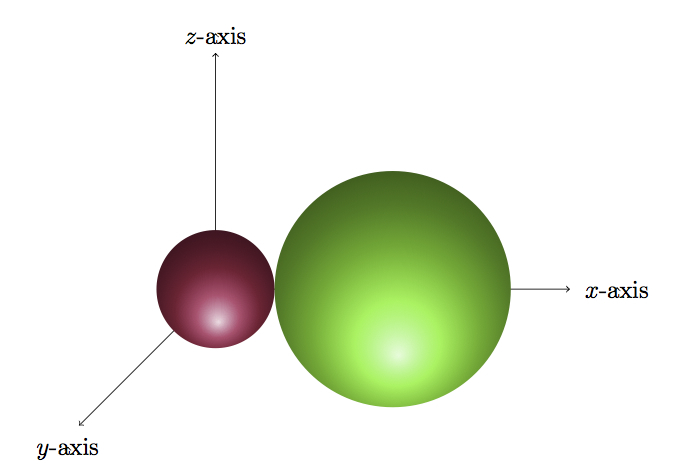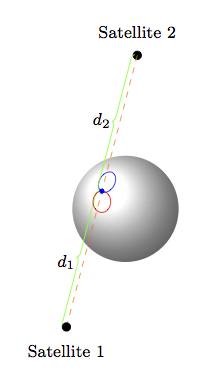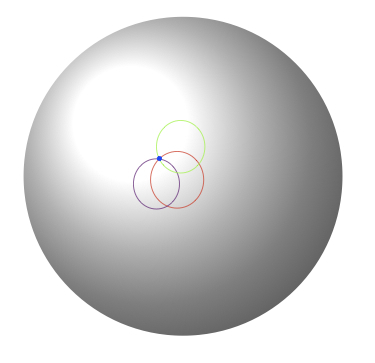# Global Positioning System II

Alignments to Content Standards: G-MG.A.1 G-GMD.B.4

Global Positioning System or GPS devices receive input from satellites and use this information to locate our position on the planet. The information received from each individual satellite gives the distance from the GPS device to that satellite and the location of the satellite. The set of points at a fixed distance from a satellite form a sphere so when the GPS receives its distance from a given satellite, this tells us that it lies on a particular sphere. Data from several satellites will locate the GPS device on the intersection of spheres. This problem examines different scenarios for intersections of spheres from the point of view of the GPS device: how many different satellites are needed to locate the GPS device? Does it matter how the satellites are configured in space?

Below is a sample picture showing three satellites and their distances, $d_1, d_2, d_3$ from a point on the earth:The three parts of this problem investigate different positions of the three satellites pictured above: the goal is to find scenarios where one, two or three of the distances $d_1$, $d_2$, $d_3$ are (or are not) sufficient to locate the GPS unit.

1. Give an example of a satellite and GPS location so that the GPS device can determine its location on the earth with input from this single satellite. Also give an example when this one signal does not provide enough information to designate a location on the earth.
2. Give an example of a configuration of two satellites and the GPS so that the GPS device can determine its location on the earth with input from the two satellites (but not from either alone). Also give an example where these two signals do not provide enough information to designate a location on the earth.
3. Give an example of a configuration of three satellites and the GPS so that the GPS device can determine its location on the earth with input from three satellites (but not from any pair of the satellites). Also give an example where three signals do not provide enough information to designate a location on the earth.

## IM Commentary

Reflective of the modernness of the technology involved, this is a challenging geometric modelling task in which students discover from scratch the geometric principles underlying the software used by GPS systems.

The geometric starting point is the observation that the set of points at a distance $d$ from a point $P$ in space (which in this problem will model the satellite) is a sphere. The earth is also modeled by a sphere for this problem, and so the key geometric objects determining the success of the GPS locator are intersections of sphere. As such, students need some familiarity with these objects (e.g., as presented in ''Global Positioning System I''). So they will need to know that an intersection of two different spheres can either be empty, a point, or a circle and this problem gives them an opportunity to apply this knowledge in a concrete setting. In particular, students will need to determine possible positions of satellites so that information from one, two, or three of them is sufficient (or not sufficient) to locate the GPS unit on the surface of the earth. This requires good geometric intuition, experimentation, and possibly dealing with the algebraic equations of the spheres involved. More information about the precise configuration of the satellites used in the Global Positioning Sytem is provided here:

From a practical perspective, unless the satellites are in very special position, readings from three satellites will suffice to locate the GPS unit on the surface of the earth (assuming that the earth is modeled as a fourth sphere). Rather than using readings from distinct satellites, in principle the GPS could receive several readings, spread over a small span of time, from a single satellite. One reason data from multiple satellites might be better is that if, for example, one of the distance readings is inaccurate, then the data will be inconsistent and the unit will have to wait for more information (or throw out the spurious reading if it is possible to identify it as such). If the data is coming from a single satellite, however, over a very short span of time, then it is possible that all readings would be similarly faulty and then the GPS unit could record the wrong position on the earth. In other words, receiving data from several satellites makes the system more robust.

GPS systems are common now on cars as well as some mobile phones. They help people navigate the world and help lost or injured people such as hikers or skiers to be found. GPS devices track time in addition to location but this has been left out of the scenario considered here. Much more information about the Global Positioning System is available at:

The earth is only approximated by a sphere but the mathematics behind this task applies to the way GPS systems actually function: thinking of the earth, in part (c) of the question, as a fourth sphere, the reasoning gives an example where these four spheres meet in exactly one point, namely the location of the GPS device. So in practice instead of using the earth as a fourth sphere, a GPS system requires a fourth satellite reading in order to determine a position in space (which happens to lie on the earth's surface).

This task allows students to develop their visual intuition while working in an important real world situation. The algebra involved in solving the modeling for different situations is rich and relatively involved. The teacher will have to make a decision as to how much of this to include along with the visualization. This is presented serpatedly in ''Global Positioning System I'' but some of this has been repeated in the solution here for convenience.

Although information from three satellites is not in general enough to locate the GPS the situations where it fails are very specific (namely, the three satellites and the center of the earth have to be coplanar) and extremely unlikely to occur. Of course there is no harm in receiving in put from four or more satellites since this will not influence the determination of the location of the GPS.

## Solutions

Solution: 1 Geometric Visualization

There are two vital aspects to this task: first visualizing the intersections of spheres and then working with equations to check that the visual intuition is correct. The GPS unit measures its distance from a given satellite. The set of all points of distance $d$ from a satellite is a sphere of radius $d$. If the earth is modeled as a sphere, the intersection of those two spheres can be a point or a circle. The case of a point is pictured below (the point at which they meet is on the $x$-axis:Another possibility is that the two spheres meet in a circle: this is pictured below (here the second sphere has been removed from the picture to show the intersection: the second sphere is located directly underneath the green sphere):A circle and a point are the only possible intersections of the sphere representing the earth and the sphere representing points on the surface of the earth a distance $d$ from the satellite: indeed, we know that there is at least one point on the earth at a distance $d$ from the satellite, namely the location of the GPS unit. On the other hand, the satellite is in outer space and so the intersection of the two spheres cannot be all of the earth.

With this information in mind, we can now give examples of the different scenarios presented in this problem.

1. This case is represented by the first picture above if we let the purple sphere represent the earth and the green sphere the set of points of distance $d$ from the satellite (so the satellite is located at the center of the green sphere). In words, if we imagine that the GPS unit is at the north pole and that the satellite is directly above the earth on the axis going through north and south poles, then the location of the GPS unit would be the only point on the earth of distance $d$ from this satellite.

In general, as in the second picture above, the set of points on the earth a distance $d$ from a satellite is not a single point but a circle as is represented in the following picture:2. If the information from either satellite alone is not enough to determine the location of the GPS this means that points of distance $d_1$ from Satellite 1 and distance $d_2$ from Satellite 2 must both be circles. So we are looking for circles on the earth which meet in a single point. This situation is captured in the picture below (the location of Satellite 2 here is a 180 degree rotation of Satellite 1 through the axis going through the center of the earth and the location of the GPS unit):Generally two circles on the surface of the earth could meet in two points rather than one and this situation is pictured below:3. Here we want a situation where the two circles on the earth representing points of distance $d_1$ from Satellite 1 and $d_2$ from Satellite 2 meet in more than one point. It is possible for them to meet in two points as is pictured here:Choosing a third satellite appropriately then identifies the right location on the earth (the satellites are not pictured here, only the points of fixed distances on the earth from those satellites):A bad situation where three satellites are not enough to determine the location of the GPS on the earth would happen, for example, if all three satellites are lined up directly over the north pole but the GPS is not at the north pole. In this situation, all three circles on the earth coming from the distances from the satellites are identical.

Solution: 2 Algebraic Equations

Here we show algebraically, with the examples used to generate the first two pictures in the first solution, why the intersection of two spheres can be a point or a circle. Then we carry out further algebraic manipulations for part (a). For parts (b) and (c) these equations become more complicated and present a formidable challenge: for part (b) we study a special case and similarly for part (c).

We begin by performing algebraic manipulations on the first two pictures given in the first solution. For the first picture, the two spheres defined by the equations $x^2 + y^2 + z^2 = 1$ and $(x-3)^2 + y^2 + z^2 = 4$. Subtracting the first equation from the second gives $(x-3)^2 - x^2 = 3$. Expanding, this becomes $-6x + 9 = 3$. This means that $x = 1$ and plugging this back into either of the equations we find that the point of intersection of the two spheres is $(1,0,0)$.

For the second picture in the first solution, consider the two spheres given by the equations $x^2 + y^2 + z^2 = 1$ and $x^2 + y^2 + \left(z - \frac{3}{2}\right)^2 = 1$. To solve these equations we can subtract the first from the second to find $$\left(z - \frac{3}{2}\right)^2 - z^2 = 0.$$ Simplifying this gives $-3z + \frac{9}{4} = 0$ so $z = \frac{3}{4}$. Plugging this into the first equation we find that the common solutions for the two equations satisfy $x^2 + y^2 = \frac{7}{16}$. This is a circle contained in the plane where $z = \frac{3}{4}$.

1. In general a signal from a single satellite will not determine a unique position on the earth but rather a circle of possible locations. There is one exception, however, namely when the satellite is directly overhead from the GPS unit. To see what this looks like in equations, suppose we model the surface of the earth by the sphere $$x^2 + y^2 + z^2 = 1.$$ Let's suppose that the GPS is at the north pole $(0,0,1)$. A point directly overhead of the north pole would be $(0,0,2)$ for example. If a satellite is located here then the GPS is one unit directly south of the satellite and the sphere of radius one centered at $(0,0,2)$ has equation $$x^2 + y^2 + (z-2)^2 = 1.$$ The only common solution for these equations is $(0,0,1)$ as can be seen by subtracting the first equation from the second givine $(z-2)^2 - z^2 = 0$ whose only solution is $z = 1$. So when the satellite is directly overhead, it is possible to determine the position on the earth from a single reading.

To see what happens when the satellite is not directly overhead, let's assume that the satellite is directly over the north pole $(0,0,1)$ but the GPS is at some other point $P$ in the northern hemisphere. Suppose the satellite has coordinates $(0,0,a)$ for some number $a$ which is larger than $1$. So the distance of the satellite to the earth is $a -1$. If $b$ denotes the distance of the satellite to the GPS then $b \gt a - 1$.

We are interested in finding the intersection of the sphere $$x^2 + y^2 + (z-a)^2 = b^2$$ with the sphere $x^2 + y^2 + z^2 = 1$: this will tell us which points on the earth are a distance $b$ from the satellite. Subtracting $x^2 + y^2 + z^2 = 1$ from this equation we find $$(z-a)^2 - z^2 = b^2 - 1.$$ This means that $-2az + a^2 = b^2 -1$ or $$z = \frac{1 + a^2 - b^2}{2a}.$$ We know this quantity is positive and is less than $1$ because the $z$ coordinate of the $GPS$ is positive (since it is in the northern hemisphere) and less than one (since it is not at the north pole). Since we also want $x^2 + y^2 + z^2 = 1$ this means that $$x^2 + y^2 = 1 - \left( \frac{1 + a^2 - b^2}{2a}\right)^2.$$ Thus the intersection of the two spheres is a circle. Moreover, this circle is contained in the plane perpendicular to the line joining the satellite and the center of the earth.

2. Suppose we continue to model the earth with the sphere $x^2 + y^2 + z^2 = 1$ and we place Satellite 1 at $(0,0,2)$ and the GPS unit at $\left(0,\frac{\sqrt{7}}{4} ,\frac{3}{4}\right)$. If we place the second satellite on the $x$-axis at $(2,0,0)$ then the points on the surface of the earth which are the same distance from Satellite 2 as the GPS unit lie on the great circle where $x = 0$, that is they are the points of the form $(0,a,b)$ where $a^2 + b^2 = 1$ (from the point of view of the previous paragraph, the plane perpendicular to the line joining $(2,0,0)$ and $(0,0,0)$ is the plane where $x = 0$). Satellite 1 tells us the longitude of the GPS unit. Satellite 2 tells us the latitude of the GPS unit except that latitudes of $x$ (where $0 \leq x \lt 180$) and $180 + x$ are both allowed. So the information from these two GPS units limits the location of the GPS unit to two possible locations: in this case those two possible locations are $\left(0,\frac{\sqrt{7}}{4} ,\frac{3}{4}\right)$ and $\left(0,\frac{-\sqrt{7}}{4} ,\frac{-3}{4}\right)$.

3. Any location of satellite 3 which will is not equidistant from the two points from part (b) will suffice. For example we could place satellite 3 at $(0,2,0)$.
4. Summarizing the two solutions, the typical situation where the information from three satellites is not enough to locate the GPS would be when the three satellites lie in a line. If they all lie on a line through the center of the earth then the distances from the respective satellites all give the same information and so they will only determine a circle of possible locations for the GPS, unless they are all directly overhead. If the three satellites lie on a plane which goes through the center of the earth then again, unless one of them lies directly overhead, it will not be possible to determine where the GPS is.

Rephrasing the previous paragraph, as long as satellites 1 and 2 have been chosen to limit the possible locations of the GPS to two points $P_1$ and $P_2$, satellite 3 can be selected to be anywhere as long as it is not equidistant from $P_1$ and $P_2$. The set of points equidistant from $P_1$ and $P_2$ forms a plane which contains satellites 1 and 2 and the center of the earth. So it is sufficient to avoid this plane and then satellite 3 will determine the location of the GPS. This is what will usually happen if three locations are chosen at random for the three satellites.